# Arithmetic progression + addition - math problems

#### Number of problems found: 41

• In the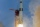In the arithmetic sequence a1 = 4.8, d = 0.4. How many consecutive members, starting with the first, need to be added so that the sum is greater than 170?
• Saving in JanuaryOn the 1st of January a students puts \$10 in a box. On the 2nd she puts \$20 in the box, and so on, putting the same number of 10-dollers notes as the day of the month. How much money will be in the box if she keeps doing this for a the first 10 days of Ja
• CalculateCalculate the sum of all three-digit natural numbers divisible by five.
• Squirrels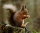The squirrels discovered a bush with hazelnuts. The first squirrel plucked one nut, the second squirrel two nuts, the third squirrel three nuts. Each new squirrel always tore one nut more than the previous squirrel. When they plucked all the nuts from the
• Common differenceThe 4th term of an arithmetic progression is 6. If the sum of the 8th and 9th terms is -72, find the common difference.
• Angles of a hexagon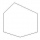Find the interior angles of a hexagon if the sizes of the angles form an arithmetic sequence, and the smallest angle is 70°.
• Sum 1-6Find the sum of the geometric progression 3, 15, 75,… to six terms.
• Insert 4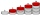Insert three arithmetic means between 3 and 63.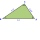The bottom rung of a ladder is 36 inches long and the topmost rung is 24 inches long. If the ladder has 18 rungs, how many inches each other rung is shorter than the rung below it. How many feet of wood was used in making the rungs?
• Sum of odd numbersFind the sum of all odd integers from 13 to 781.
• 1 pageOne page is torn from the book. The sum of the page numbers of all the remaining pages is 15,000. What numbers did the pages have on the page that was torn from the book?
• Finite arithmetic sequenceHow many numbers should be inserted between the numbers 1 and 25 so that all numbers create a finite arithmetic sequence and that the sum of all members of this group is 117?
• Sum of the seventeen numbersThe sum of the 17 different natural numbers is 154. Determine the sum of the two largest ones.
• Sit-ups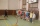Vojta strengthens for 8 weeks. In the first week he did 5 sit-ups a day. Every next week he did 1 more per day than in the previous week. How many sit-ups has Vojta made?
• The sumThe sum of the first 10 members of the arithmetic sequence is 120. What will be the sum if the difference is reduced by 2?
• Pine's forest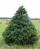There were so many pines in the forest that they were sequentially numbered 1, 2, 3,..., would use three times more digits than the pine trees alone. How many pine trees were there in the forest?
• Bonuses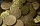Five employees of the company were paid bonuses so that each successor received 550 USD less than the previous employee. How much did everyone get if a total of USD 11,000 has paid?
• Loan 5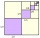Abdul takes a loan of 200000 from Ali and agrees to repay in number of instalment, each instalment begin with the 2nd exceeding the previous one by 1000, if the first instalment is 500, find how many instalment will be necessary to be wipe out the loan? C
• Find the sumFind the sum of all natural numbers from 1 and 100, which are divisible by 2 or 5
• Infinite sum of areasAbove the height of the equilateral triangle ABC is constructed an equilateral triangle A1, B1, C1, of the height of the equilateral triangle built A2, B2, C2, and so on. The procedure is repeated continuously. What is the total sum of the areas of all tr

Do you have an exciting math question or word problem that you can't solve? Ask a question or post a math problem, and we can try to solve it.

We will send a solution to your e-mail address. Solved examples are also published here. Please enter the e-mail correctly and check whether you don't have a full mailbox.

Please do not submit problems from current active competitions such as Mathematical Olympiad, correspondence seminars etc...

Arithmetic progression - math problems. Addition Problems.